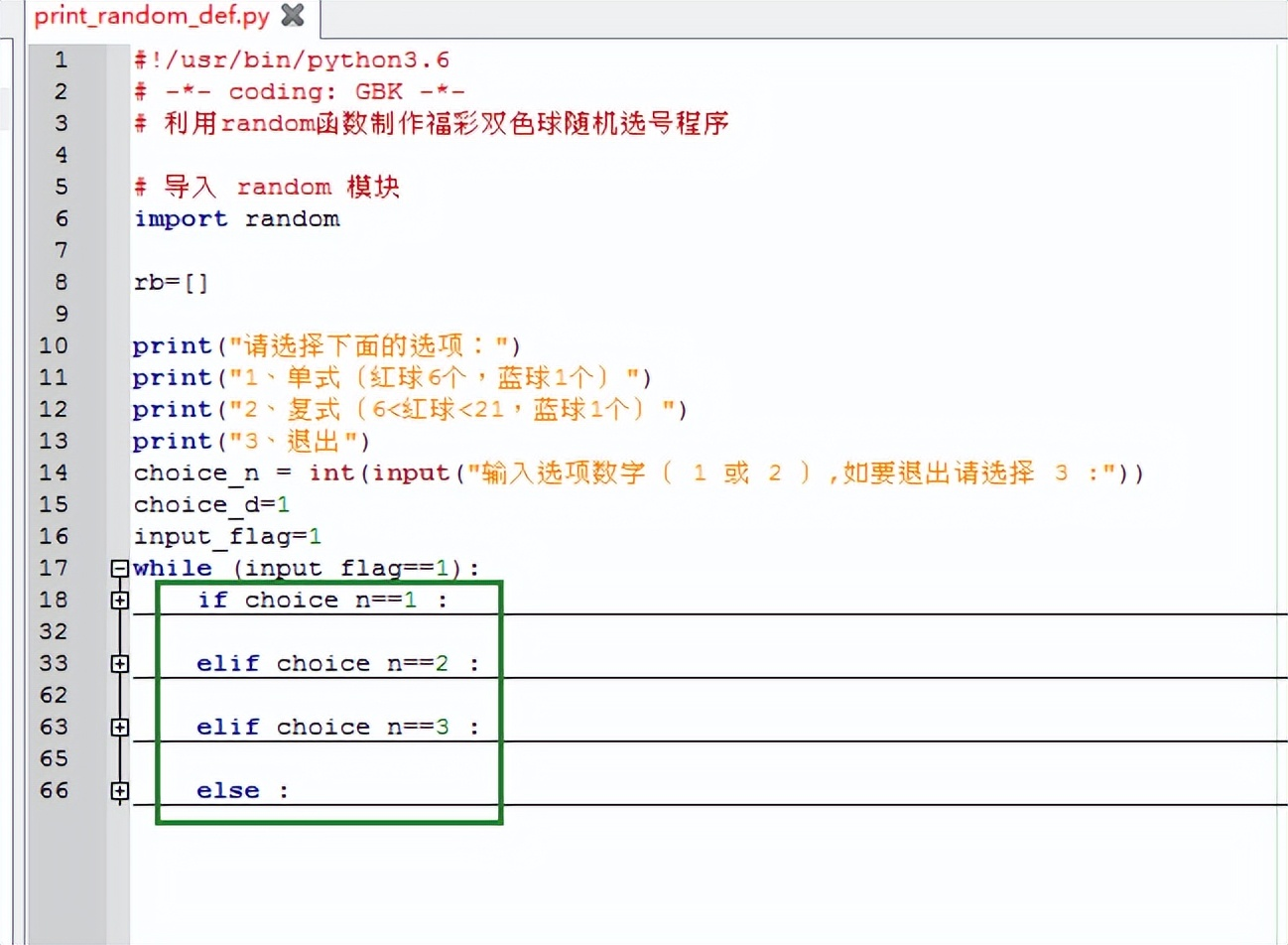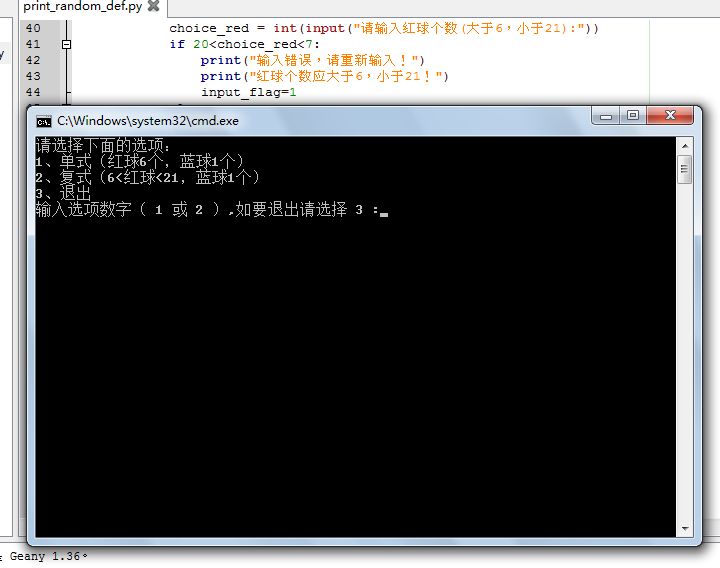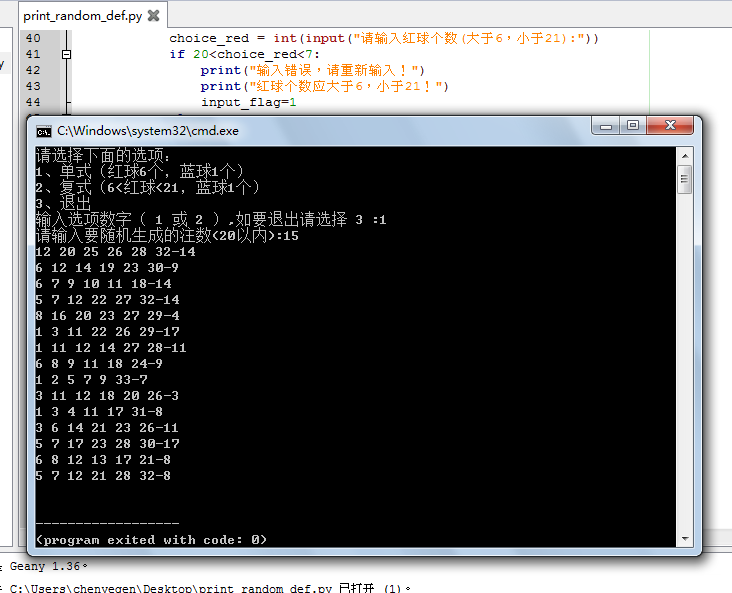# Python程序开发之简单小程序实例（7）双色球随机选号程序

Python程序开发之简单小程序实例

（7）双色球随机选号程序

#!/usr/bin/python3.6

# -*- coding: GBK -*-

# 利用random函数制作福彩双色球随机选号程序

# 导入 random 模块

import random

rb=[]

print("请选择下面的选项：")

print("1、单式（红球6个，蓝球1个）")

print("2、复式（6<红球<21，蓝球1个）")

print("3、退出")

choice_n = int(input("输入选项数字（ 1 或 2 ）,如要退出请选择 3 :"))

choice_d=1

input_flag=1

while (input_flag==1):

if choice_n==1 :

choice_d = int(input("请输入要随机生成的注数(20以内):"))

if choice_d==0 or choice_d>20:

print("输入错误，请重新输入！")

print("请输入大于0，小于等于20的数字！")

input_flag=1

else :

for i in range(1,choice_d+1):

rb=random.sample(range(1, 34), 6)

rb.sort()

rb_blue=random.randint(1, 17)

rb_str=str(rb)+" "+str(rb)+" "+str(rb)+" "+str(rb)+" "+str(rb)+" "+str(rb)+"-"+str(rb_blue)

print(rb_str)

break

elif choice_n==2 :

choice_d = int(input("请输入要随机生成的注数(20以内):"))

if choice_d==0 or choice_d>20:

print("输入错误，请重新输入！")

print("请输入大于0，小于等于20的数字！")

input_flag=1

else :

choice_red = int(input("请输入红球个数(大于6，小于21):"))

if 20<choice_red<7:

print("输入错误，请重新输入！")

print("红球个数应大于6，小于21！")

input_flag=1

else :

for i in range(1,choice_d+1):

rb=random.sample(range(1, 34), choice_red+1)

rb.sort()

rb_blue=random.randint(1, 17)

for j in range(0,choice_red):

if j==0:

rb_str=str(rb[j])+" "

elif j==choice_red-1 :

rb_str=rb_str+str(rb[j])

else :

rb_str=rb_str+str(rb[j])+" "

rb_str=rb_str+"-"+str(rb_blue)

print(rb_str)

break

elif choice_n==3 :

break

else :

print("输入错误，请重新输入！")

choice_n = int(input("输入选项数字（ 1 或 2 ）,如要退出请选择 3 :"))

input_flag=11、提示用户选择功能2、根据用户输入完成相应功能：